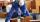Garden

Father dig up the garden in 9 hours. Son in 13 hours. How many hours take dig up the garden together?

Result

x =  5.318 h

Solution:

x/9+x/13 = 1

22x = 117

x = 11722 ≈ 5.318182

Calculated by our simple equation calculator.

Leave us a comment of example and its solution (i.e. if it is still somewhat unclear...):Be the first to comment!To solve this verbal math problem are needed these knowledge from mathematics:

Looking for help with calculating harmonic mean? Looking for a statistical calculator? Need help calculate sum, simplify or multiply fractions? Try our fraction calculator. Do you have a linear equation or system of equations and looking for its solution? Or do you have quadratic equation?

Next similar examples:

1. TogetherGrandfather, father, and son are carpenters. They decided to make a wardrobe. Father produces it for 12 hours, his son for 15 hours, and grandfather for 10 hours. How long will they make it together?
2. SoldierAn experienced soldier digs a good trench in 30 hours. Two soldiers dug the same trench each 40 hours. How many hours will the ditch dig all three together?
3. In fractionsAn ant climbs 2/5 of the pole on the first hour and climbs 1/4 of the pole on the next hour. What part of the pole does the ant climb in two hours?
4. Boys and girlsThere are 28 girls in the hall. 5/7 of all children are boys. How many children and how many boys are there?
5. CakesGrandmother baked cakes. Half of its was poppy, quarter with plum jam and 16 cheesecakes. How many cakes she baked in total?
6. Bricklayers8 bricklayers build a house for 630 days. How many bricklayers have to add after 150 days, so then the whole building completed in (next) 320 days?
7. Three paintersThe three painters have painted bridge. The first would work done in 5 days, the second in 6 days, and the third in 7.5 days. How long will the bridge work if they work together?
8. Find xSolve: if 2(x-1)=14, then x= (solve an equation with one unknown)
9. Unknown number 6Determine x if 1/6 of x is equal to 2/5 of the number 24.
10. One-thirdA one-third of unknown number is equal to five times as great as the difference of the same unknown number and number 28. Determine the unknown number.
11. FractionsThree-quarters of an unknown number are 4/5. What is 5/6 of this unknown number?
12. Equation 15Solve equation with variables on both sides:
13. EquationSolve equation and check the result: 1.4x - 3/2 + x - 9,8 = x + 0,4/3 - 7 + 1,6/6
14. Equation 29Solve next equation: 2 ( 2x + 3 ) = 8 ( 1 - x) -5 ( x -2 )
15. SimplifySimplify the following problem and express as a decimal: 5.68-[5-(2.69+5.65-3.89) /0.5]
16. HotelThe hotel has a p floors each floor has i rooms from which the third are single and the others are double. Represents the number of beds in hotel.
17. Fraction to decimalWrite the fraction 3/22 as a decimal.